# Team:UANL Mty-Mexico/Workshop Math

Carousel Template for Bootstrap

### Math Modelling Course

This year, the iGEM UANL team decided to establish an introductory course called 'Mathematical Modeling in Systems Biology', with the idea of preparing our faculty students in the mathematical area of biology.

This course is made up of three phases, each one demanding a different knowledge level.

Phase I Has the intention of teaching the students theoretical and applicable aspects of differential and integral calculus as a fundamental part in solving and distinguishing differential equations.

Phase II Was designed with the aim of making the students able to understand the applications of linear transformation of vector spaces for the solution of differential equations that can be applied in systems biology.

Phase III. The objective of this part of the course is that students come to understand the general concepts of systems biology and become familiar with its theoretical basis, having as purpose increasing the student's critical awareness on this area.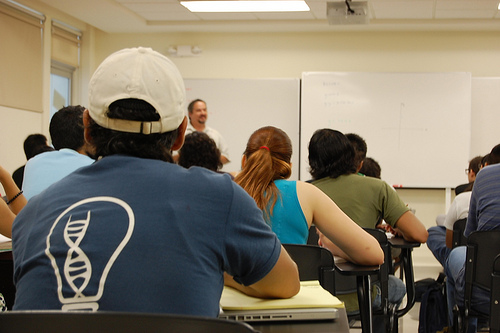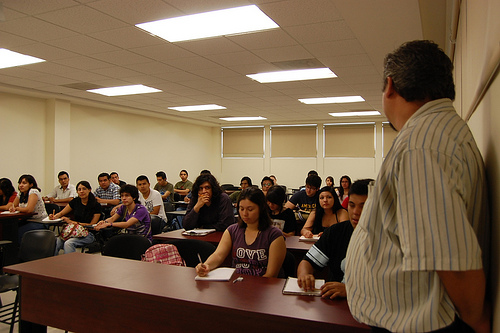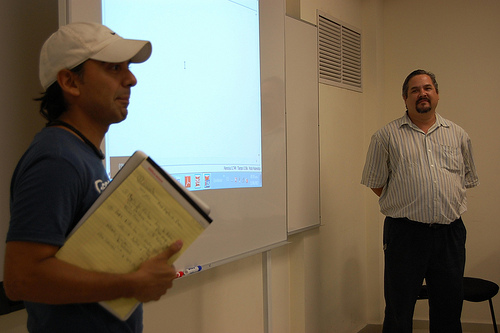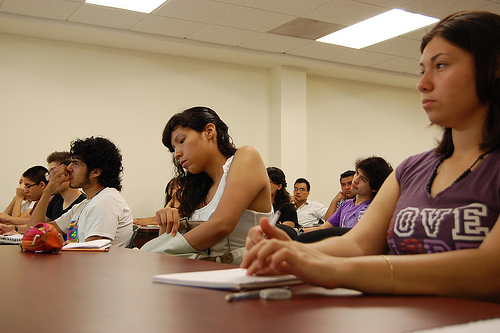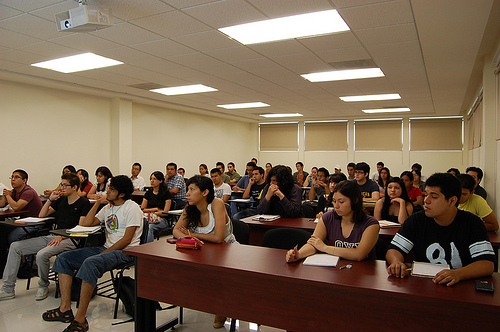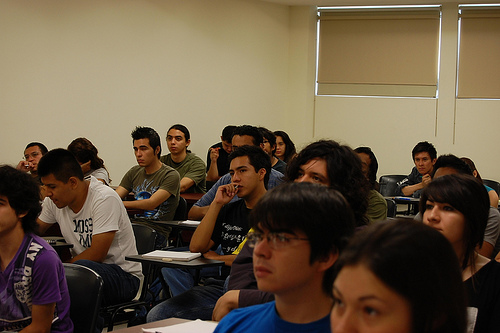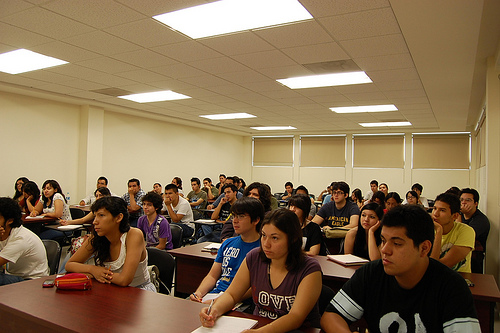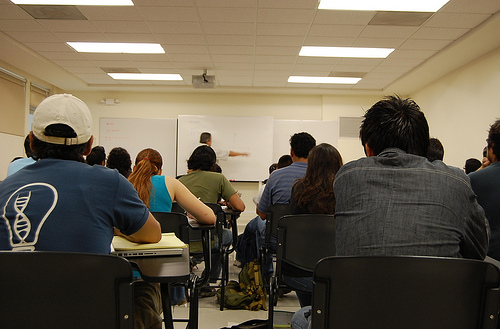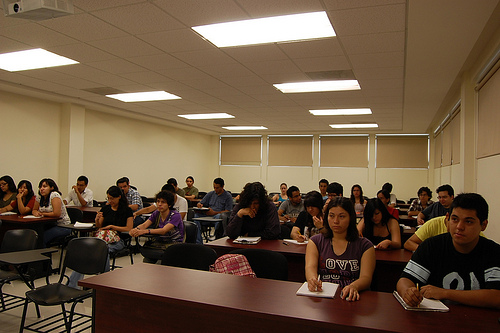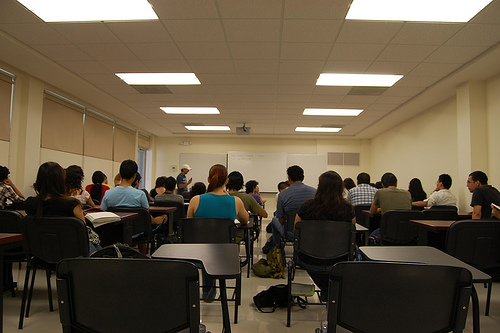## Description

This program is based on the UNAM Genomic Sciences career's syllabus, which is an internationally-recognised career by many other universities and prestigious investigation centers in the world.

The first phase of the course was successfully imparted along June and July of this year by M.Sc. Jesús Botello González, professor of the Chemistry School of our University (UANL). It had a duration of 6 weeks and took place in our faculty; approximately 40 students enrolled this course.

M.Sc. Botello will impart phase II as well, but the program has still to be dated.

We think this course is a great step toward the learning of advanced knowledge in the field of molecular biology and genomics that has to be made use of, and it is really important for the UANL students, so that we are well-educated in this field.

The subjects that are included in the first phase (Phase I) of this course are:

1. Precalculus

1.1 Elementary algebra
1.2 Understanding of the behavior of functions through their graphs.
1.3 Functions, dependent and independent variables, domain and range.
1.4 Function composition
1.5 Inverse functions

2. Differential calculus

2.1 Limits
2.2 Lateral limits
2.3 Infinite limits
2.4 Limits tending to infinite
2.5 Behavior of asymptotic rational functions applying limits
2.6 Continuity
2.7 Definition of derivatives
2.8 Derivation rules
2.9 Derivative of a composite function
2.10 Chain rule
2.11 Change rate in relation to time
2.12 Optimization
2.13 Function graphing using derivatives

3. Integral calculus

3.1 Sigma notation
3.2 Riemann sums (Definite integral definition)
3.3 Calculation of the area under a curve with definite integrals
3.4 Integral calculus theorems, including the Fundamental Theorem of Calculus
3.5 Definition of indefinite integrals (anti-derivatives)
3.6 Polynomial function integration
3.7 Integration by substitution
3.8 Calculation of the area between functions
3.9 Calculation of volume by slices
3.10 Calculation of the volume of solids of revolution with discs and cylindric layers
3.11 Integration techniques